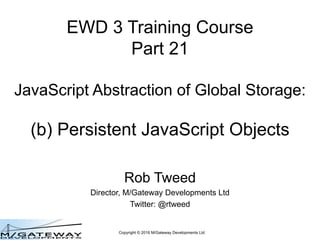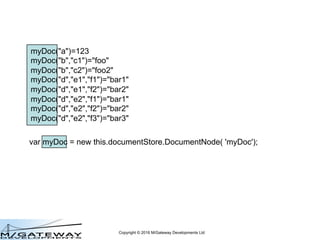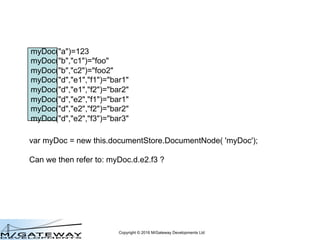Successfully reported this slideshow.

# EWD 3 Training Course Part 21: Persistent JavaScript Objects

2

Share×
1 of 31
1 of 31

# EWD 3 Training Course Part 21: Persistent JavaScript Objects

2

Share

This presentation is Part 21 of the EWD 3 Training Course. It explains how Document Node objects and its \$() function allow the abstraction of Persistent JavaScript Objects from Global Storage

This presentation is Part 21 of the EWD 3 Training Course. It explains how Document Node objects and its \$() function allow the abstraction of Persistent JavaScript Objects from Global Storage

## More Related Content

### Related Books

Free with a 14 day trial from Scribd

See all

### Related Audiobooks

Free with a 14 day trial from Scribd

See all

### EWD 3 Training Course Part 21: Persistent JavaScript Objects

1. 1. Copyright © 2016 M/Gateway Developments Ltd EWD 3 Training Course Part 21 JavaScript Abstraction of Global Storage: (b) Persistent JavaScript Objects Rob Tweed Director, M/Gateway Developments Ltd Twitter: @rtweed
2. 2. Copyright © 2016 M/Gateway Developments Ltd var myDoc = new this.documentStore.DocumentNode( 'myDoc'); myDoc("a")=123 myDoc("b","c1")="foo" myDoc("b","c2")="foo2" myDoc("d","e1","f1")="bar1" myDoc("d","e1","f2")="bar2" myDoc("d","e2","f1")="bar1" myDoc("d","e2","f2")="bar2" myDoc("d","e2","f3")="bar3"
3. 3. Copyright © 2016 M/Gateway Developments Ltd var myDoc = new this.documentStore.DocumentNode( 'myDoc'); Can we then refer to: myDoc.d.e2.f3 ? myDoc("a")=123 myDoc("b","c1")="foo" myDoc("b","c2")="foo2" myDoc("d","e1","f1")="bar1" myDoc("d","e1","f2")="bar2" myDoc("d","e2","f1")="bar1" myDoc("d","e2","f2")="bar2" myDoc("d","e2","f3")="bar3"
4. 4. Copyright © 2016 M/Gateway Developments Ltd var myDoc = new this.documentStore.DocumentNode( 'myDoc'); myDoc.d.e2.f3 Is a DocumentNode Object myDoc("a")=123 myDoc("b","c1")="foo" myDoc("b","c2")="foo2" myDoc("d","e1","f1")="bar1" myDoc("d","e1","f2")="bar2" myDoc("d","e2","f1")="bar1" myDoc("d","e2","f2")="bar2" myDoc("d","e2","f3")="bar3"
5. 5. Copyright © 2016 M/Gateway Developments Ltd var myDoc = new this.documentStore.DocumentNode( 'myDoc'); myDoc.d.e2.f3 myDoc("a")=123 myDoc("b","c1")="foo" myDoc("b","c2")="foo2" myDoc("d","e1","f1")="bar1" myDoc("d","e1","f2")="bar2" myDoc("d","e2","f1")="bar1" myDoc("d","e2","f2")="bar2" myDoc("d","e2","f3")="bar3" Would also have to be a DocumentNode Object This would mean we'd have to know that the 'd' property was, or could be, a valid node
6. 6. Copyright © 2016 M/Gateway Developments Ltd var myDoc = new this.documentStore.DocumentNode( 'myDoc'); myDoc.d.e2.f3 myDoc("a")=123 myDoc("b","c1")="foo" myDoc("b","c2")="foo2" myDoc("d","e1","f1")="bar1" myDoc("d","e1","f2")="bar2" myDoc("d","e2","f1")="bar1" myDoc("d","e2","f2")="bar2" myDoc("d","e2","f3")="bar3" Would also have to be a DocumentNode Object
7. 7. Copyright © 2016 M/Gateway Developments Ltd var myDoc = new this.documentStore.DocumentNode( 'myDoc'); myDoc.d.e2.f3 myDoc("a")=123 myDoc("b","c1")="foo" myDoc("b","c2")="foo2" myDoc("d","e1","f1")="bar1" myDoc("d","e1","f2")="bar2" myDoc("d","e2","f1")="bar1" myDoc("d","e2","f2")="bar2" myDoc("d","e2","f3")="bar3" Would also have to be a DocumentNode Object
8. 8. Copyright © 2016 M/Gateway Developments Ltd var myDoc = new this.documentStore.DocumentNode( 'myDoc'); myDoc.d.e2.f3 BUT: Impossible to predict or determine in advance all the possible subscripts of a Global myDoc("a")=123 myDoc("b","c1")="foo" myDoc("b","c2")="foo2" myDoc("d","e1","f1")="bar1" myDoc("d","e1","f2")="bar2" myDoc("d","e2","f1")="bar1" myDoc("d","e2","f2")="bar2" myDoc("d","e2","f3")="bar3"
9. 9. Copyright © 2016 M/Gateway Developments Ltd var myDoc = new this.documentStore.DocumentNode( 'myDoc'); myDoc.d.e2.f3 BUT: Impossible to predict or determine in advance all the possible subscripts of a Global •No schema to tell us myDoc("a")=123 myDoc("b","c1")="foo" myDoc("b","c2")="foo2" myDoc("d","e1","f1")="bar1" myDoc("d","e1","f2")="bar2" myDoc("d","e2","f1")="bar1" myDoc("d","e2","f2")="bar2" myDoc("d","e2","f3")="bar3"
10. 10. Copyright © 2016 M/Gateway Developments Ltd var myDoc = new this.documentStore.DocumentNode( 'myDoc'); myDoc.d.e2.f3 BUT: Impossible to predict or determine in advance all the possible subscripts of a Global •No schema to tell us •could be hundreds of thousands, or millions of subscript values and any number of levels of hierarchy myDoc("a")=123 myDoc("b","c1")="foo" myDoc("b","c2")="foo2" myDoc("d","e1","f1")="bar1" myDoc("d","e1","f2")="bar2" myDoc("d","e2","f1")="bar1" myDoc("d","e2","f2")="bar2" myDoc("d","e2","f3")="bar3"
11. 11. Copyright © 2016 M/Gateway Developments Ltd var myDoc = new this.documentStore.DocumentNode( 'myDoc'); myDoc.x.value Also: can't create properties automatically using subscript names as these might clash with DocumentNode property or method names as in the case above myDoc("a")=123 myDoc("b","c1")="foo" myDoc("b","c2")="foo2" myDoc("d","e1","f1")="bar1" myDoc("d","e1","f2")="bar2" myDoc("d","e2","f1")="bar1" myDoc("d","e2","f2")="bar2" myDoc("d","e2","f3")="bar3" myDoc("x","value")="hello world"
12. 12. Copyright © 2016 M/Gateway Developments Ltd \$() Function var myDoc = new this.documentStore.DocumentNode('myDoc'); var dNode = myDoc.\$('d'); myDoc("a")=123 myDoc("b","c1")="foo" myDoc("b","c2")="foo2" myDoc("d","e1","f1")="bar1" myDoc("d","e1","f2")="bar2" myDoc("d","e2","f1")="bar1" myDoc("d","e2","f2")="bar2" myDoc("d","e2","f3")="bar3"
13. 13. Copyright © 2016 M/Gateway Developments Ltd \$() Function var myDoc = new this.documentStore.DocumentNode('myDoc'); var dNode = myDoc.\$('d'); myDoc("a")=123 myDoc("b","c1")="foo" myDoc("b","c2")="foo2" myDoc("d","e1","f1")="bar1" myDoc("d","e1","f2")="bar2" myDoc("d","e2","f1")="bar1" myDoc("d","e2","f2")="bar2" myDoc("d","e2","f3")="bar3"
14. 14. Copyright © 2016 M/Gateway Developments Ltd \$() Function var myDoc = new this.documentStore.DocumentNode('myDoc'); var dNode = myDoc.\$('d'); myDoc("a")=123 myDoc("b","c1")="foo" myDoc("b","c2")="foo2" myDoc("d","e1","f1")="bar1" myDoc("d","e1","f2")="bar2" myDoc("d","e2","f1")="bar1" myDoc("d","e2","f2")="bar2" myDoc("d","e2","f3")="bar3" \$(): - Returns a DocumentNode Object representing the specified sub-node of the parent DocumentNode
15. 15. Copyright © 2016 M/Gateway Developments Ltd \$() Function var myDoc = new this.documentStore.DocumentNode('myDoc'); var dNode = new this.documentStore.DocumentNode('myDoc', [ 'd']); This does the same thing, but is much more verbose myDoc("a")=123 myDoc("b","c1")="foo" myDoc("b","c2")="foo2" myDoc("d","e1","f1")="bar1" myDoc("d","e1","f2")="bar2" myDoc("d","e2","f1")="bar1" myDoc("d","e2","f2")="bar2" myDoc("d","e2","f3")="bar3"
16. 16. Copyright © 2016 M/Gateway Developments Ltd Chaining \$() Functions var myDoc = new this.documentStore.DocumentNode('myDoc'); var dNode = myDoc.\$('d'); myDoc("a")=123 myDoc("b","c1")="foo" myDoc("b","c2")="foo2" myDoc("d","e1","f1")="bar1" myDoc("d","e1","f2")="bar2" myDoc("d","e2","f1")="bar1" myDoc("d","e2","f2")="bar2" myDoc("d","e2","f3")="bar3"
17. 17. Copyright © 2016 M/Gateway Developments Ltd Chaining \$() Functions var myDoc = new this.documentStore.DocumentNode('myDoc'); var dNode = myDoc.\$('d'); var e2Node = dNode.\$('e2'); myDoc("a")=123 myDoc("b","c1")="foo" myDoc("b","c2")="foo2" myDoc("d","e1","f1")="bar1" myDoc("d","e1","f2")="bar2" myDoc("d","e2","f1")="bar1" myDoc("d","e2","f2")="bar2" myDoc("d","e2","f3")="bar3"
18. 18. Copyright © 2016 M/Gateway Developments Ltd Chaining \$() Functions var myDoc = new this.documentStore.DocumentNode('myDoc'); var dNode = myDoc.\$('d'); var e2Node = myDoc.\$('d').\$('e2'); Does the same thing by chaining myDoc("a")=123 myDoc("b","c1")="foo" myDoc("b","c2")="foo2" myDoc("d","e1","f1")="bar1" myDoc("d","e1","f2")="bar2" myDoc("d","e2","f1")="bar1" myDoc("d","e2","f2")="bar2" myDoc("d","e2","f3")="bar3"
19. 19. Copyright © 2016 M/Gateway Developments Ltd Chaining \$() Functions var myDoc = new this.documentStore.DocumentNode('myDoc'); var f3Node = myDoc.\$('d').\$('e2').\$('f3'); myDoc("a")=123 myDoc("b","c1")="foo" myDoc("b","c2")="foo2" myDoc("d","e1","f1")="bar1" myDoc("d","e1","f2")="bar2" myDoc("d","e2","f1")="bar1" myDoc("d","e2","f2")="bar2" myDoc("d","e2","f3")="bar3"
20. 20. Copyright © 2016 M/Gateway Developments Ltd \$() only needs invoking once var myDoc = new this.documentStore.DocumentNode('myDoc'); var f3Node = myDoc.\$('d').\$('e2').\$('f3'); myDoc("a")=123 myDoc("b","c1")="foo" myDoc("b","c2")="foo2" myDoc("d","e1","f1")="bar1" myDoc("d","e1","f2")="bar2" myDoc("d","e2","f1")="bar1" myDoc("d","e2","f2")="bar2" myDoc("d","e2","f3")="bar3" A DocumentNode represented by \$d was instantiated as a property of the myDoc DocumentNode
21. 21. Copyright © 2016 M/Gateway Developments Ltd \$() only needs invoking once var myDoc = new this.documentStore.DocumentNode('myDoc'); var f3Node = myDoc.\$('d').\$('e2').\$('f3'); myDoc("a")=123 myDoc("b","c1")="foo" myDoc("b","c2")="foo2" myDoc("d","e1","f1")="bar1" myDoc("d","e1","f2")="bar2" myDoc("d","e2","f1")="bar1" myDoc("d","e2","f2")="bar2" myDoc("d","e2","f3")="bar3" A DocumentNode represented by \$d was instantiated as a property of the myDoc DocumentNode Now accessible as myDoc.\$d
22. 22. Copyright © 2016 M/Gateway Developments Ltd \$() only needs invoking once var myDoc = new this.documentStore.DocumentNode('myDoc'); var f3Node = myDoc.\$('d').\$('e2').\$('f3'); myDoc("a")=123 myDoc("b","c1")="foo" myDoc("b","c2")="foo2" myDoc("d","e1","f1")="bar1" myDoc("d","e1","f2")="bar2" myDoc("d","e2","f1")="bar1" myDoc("d","e2","f2")="bar2" myDoc("d","e2","f3")="bar3" A DocumentNode represented by \$e2 was instantiated as a property of myDoc.\$d
23. 23. Copyright © 2016 M/Gateway Developments Ltd \$() only needs invoking once var myDoc = new this.documentStore.DocumentNode('myDoc'); var f3Node = myDoc.\$('d').\$('e2').\$('f3'); myDoc("a")=123 myDoc("b","c1")="foo" myDoc("b","c2")="foo2" myDoc("d","e1","f1")="bar1" myDoc("d","e1","f2")="bar2" myDoc("d","e2","f1")="bar1" myDoc("d","e2","f2")="bar2" myDoc("d","e2","f3")="bar3" A DocumentNode represented by \$e2 was instantiated as a property of myDoc.\$d Now accesible as myDoc.\$d.\$e2
24. 24. Copyright © 2016 M/Gateway Developments Ltd \$() only needs invoking once var myDoc = new this.documentStore.DocumentNode('myDoc'); var f3Node = myDoc.\$('d').\$('e2').\$('f3'); myDoc("a")=123 myDoc("b","c1")="foo" myDoc("b","c2")="foo2" myDoc("d","e1","f1")="bar1" myDoc("d","e1","f2")="bar2" myDoc("d","e2","f1")="bar1" myDoc("d","e2","f2")="bar2" myDoc("d","e2","f3")="bar3" Only need to use \$() once for any specific subscript
25. 25. Copyright © 2016 M/Gateway Developments Ltd \$() only needs invoking once var myDoc = new this.documentStore.DocumentNode('myDoc'); var f3Node = myDoc.\$('d').\$('e2').\$('f3'); var f2Node = myDoc.\$('d').\$('e2').\$('f2'); myDoc("a")=123 myDoc("b","c1")="foo" myDoc("b","c2")="foo2" myDoc("d","e1","f1")="bar1" myDoc("d","e1","f2")="bar2" myDoc("d","e2","f1")="bar1" myDoc("d","e2","f2")="bar2" myDoc("d","e2","f3")="bar3" So we don’t need to do this
26. 26. Copyright © 2016 M/Gateway Developments Ltd \$() only needs invoking once var myDoc = new this.documentStore.DocumentNode('myDoc'); var f3Node = myDoc.\$('d').\$('e2').\$('f3'); var f2Node = myDoc.\$d.\$e2.\$('f2'); myDoc("a")=123 myDoc("b","c1")="foo" myDoc("b","c2")="foo2" myDoc("d","e1","f1")="bar1" myDoc("d","e1","f2")="bar2" myDoc("d","e2","f1")="bar1" myDoc("d","e2","f2")="bar2" myDoc("d","e2","f3")="bar3" We can do this instead, provided \$() has been used previously for the specified subscript
27. 27. Copyright © 2016 M/Gateway Developments Ltd \$ properties are DocumentNodes var myDoc = new this.documentStore.DocumentNode('myDoc'); var f3Node = myDoc.\$('d').\$('e2').\$('f3'); var f3Value = f3Node.value; // bar3 myDoc("a")=123 myDoc("b","c1")="foo" myDoc("b","c2")="foo2" myDoc("d","e1","f1")="bar1" myDoc("d","e1","f2")="bar2" myDoc("d","e2","f1")="bar1" myDoc("d","e2","f2")="bar2" myDoc("d","e2","f3")="bar3"
28. 28. Copyright © 2016 M/Gateway Developments Ltd \$ properties are DocumentNodes var myDoc = new this.documentStore.DocumentNode('myDoc'); var f3Node = myDoc.\$('d').\$('e2').\$('f3'); var f3Value = myDoc.\$d.\$e2.\$f3.value; // bar3 myDoc("a")=123 myDoc("b","c1")="foo" myDoc("b","c2")="foo2" myDoc("d","e1","f1")="bar1" myDoc("d","e1","f2")="bar2" myDoc("d","e2","f1")="bar1" myDoc("d","e2","f2")="bar2" myDoc("d","e2","f3")="bar3"
29. 29. Copyright © 2016 M/Gateway Developments Ltd \$ properties are DocumentNodes var myDoc = new this.documentStore.DocumentNode('myDoc'); var f3Node = myDoc.\$('d').\$('e2').\$('f3'); myDoc.\$d.\$e2.\$f3.value = 'New value'; myDoc("a")=123 myDoc("b","c1")="foo" myDoc("b","c2")="foo2" myDoc("d","e1","f1")="bar1" myDoc("d","e1","f2")="bar2" myDoc("d","e2","f1")="bar1" myDoc("d","e2","f2")="bar2" myDoc("d","e2","f3")="bar3"
30. 30. Copyright © 2016 M/Gateway Developments Ltd Persistent JavaScript Objects! var myDoc = new this.documentStore.DocumentNode('myDoc'); var f3Node = myDoc.\$('d').\$('e2').\$('f3'); myDoc.\$d.\$e2.\$f3.value = 'New value'; myDoc("a")=123 myDoc("b","c1")="foo" myDoc("b","c2")="foo2" myDoc("d","e1","f1")="bar1" myDoc("d","e1","f2")="bar2" myDoc("d","e2","f1")="bar1" myDoc("d","e2","f2")="bar2" myDoc("d","e2","f3")=”New value" Updates value on disk, not an in-memory copy "Persistent JavaScript Objects"
31. 31. Copyright © 2016 M/Gateway Developments Ltd Persistent JavaScript Objects myDoc("a")=123 myDoc("b","c1")="foo" myDoc("b","c2")="foo2" myDoc("d","e1","f1")="bar1" myDoc("d","e1","f2")="bar2" myDoc("d","e2","f1")="bar1" myDoc("d","e2","f2")="bar2" myDoc("d","e2","f3")=”New value" Not quite: myDoc.d.e2.f3 Instead: myDoc.\$d.\$e2.\$f3 But no chance of subscript-based properties clashing with DocumentNode property or method names by prefixing with \$